# Generating Random Numbers From Linux TerminalGenerating random numbers is a fun kind of work to do. Sometimes, such tasks are needed for a purpose too like creating a password. In Linux, there are several ways to generate random numbers with some scripts.

In this article, we are going to explain the different ways to generate random numbers through Linux terminals.

## Use of \$RANDOM variable

One way is to use the \$RANDOM variable to generate random numbers within the range of 0 and 32767. It is a builtin shell variable to generate the random number. To create random numbers, run the command as shown below.

`\$ echo \$RANDOM`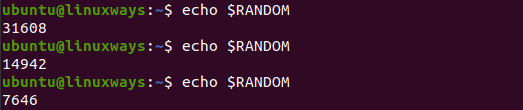## Generate random number between the chosen range

With the small tweak on a command, you are able to generate random numbers between the chosen range. To generate a random number between the range 0 to 30, you have to run the command as shown below.

`\$ a=\$(( \$RANDOM % 31 ))`
`\$ echo \$a`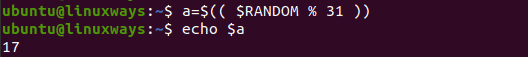Another way to generate a random number between 0 to 100, you have to run the command as shown below.

`\$ echo \$(( \$RANDOM % 101 ))`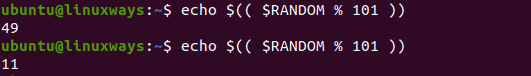## Generate random number using the script

With the use of bash scripting, random numbers can also be easily generated. It is also a bit fun to write the script for such a purpose. Simple example of a script to generate a random number is shown below.

`\$ sudo vim randomnumber.sh`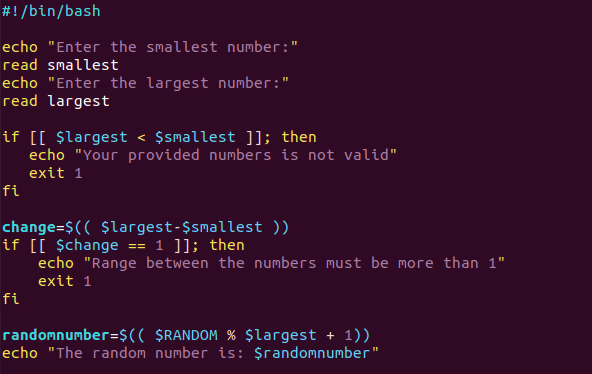```#!/bin/bash

echo "Enter the smallest number:"

echo "Enter the largest number:"

if [[ \$largest < \$smallest ]]; then

echo "Your provided numbers is not valid"

exit 1

fi

change=\$(( \$largest-\$smallest ))

if [[ \$change == 1 ]]; then

echo "Range between the numbers must be more than 1"

exit 1

fi

randomnumber=\$(( \$RANDOM % \$largest + 1))

echo "The random number is: \$randomnumber"```

Output: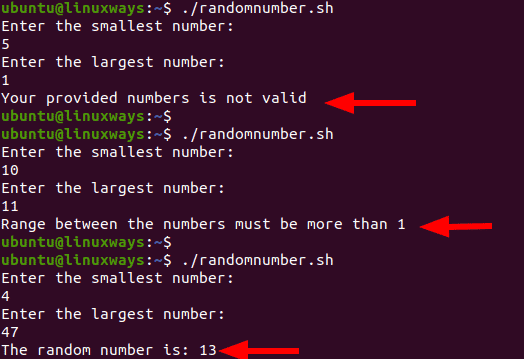With such a script, you are able to generate random numbers between the range provided by you.

## Use of shuf command

It is also one of the ways to generate random numbers between the ranges. Run a simple command on the Linux terminal for such a purpose. To generate the random number between the range 7 and 57, run the command as shown below.

`\$ shuf -i 7-57 -n1`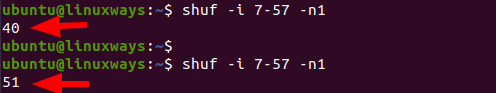### Use of tr command with urandom

To generate the random number with “n” number of digits, you can pull numbers from the /dev/urandom stream with the use of tr command. Suppose to generate a random number with 3 and 7 digits, you have to run the command as shown below.

`\$ tr -cd "[:digit:]" < /dev/urandom | head -c 3`
`\$ tr -cd "[:digit:]" < /dev/urandom | head -c 7`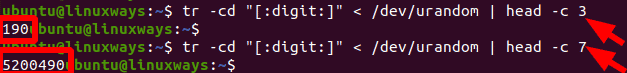## Conclusion

In this article, you have learnt to generate random numbers with a small command on the terminal or by creating the script. Also, you learnt to create random numbers between the range as provided. Thank you!